﻿ 基于分位数自回归模型的健康险保费收入研究

# 基于分位数自回归模型的健康险保费收入研究Study on the Health Insurance Premiums Income Based on Quartile Autoregression Model

Abstract:
Health insurance premium income is a key economic indicator to measure the development of health insurance industry. In this paper, the time series data of health insurance premium income from January 1991 to June 2017 were modeled with the quantile regression method. Firstly, the model AR(3) was identified, and then the quantile autoregressive model was established by the method of quantile regression, and the increase and decrease trend of the original data was fitted accurately. Finally, the short-term prediction is made by autoregressive AR(3) model and quantile autoregressive QAR(3) model respectively. The prediction results of the two models were compared based on several evaluation indicators. The results show that the quantile autoregressive model is more effective.

1. 引言

Koenker和Bassett (1978)  提出的分位数回归是最小二乘回归方法的优化  。与普通的均值回归相比，分位数回归能精细地刻画自变量对于响应变量对应于不同分位点的不同影响。将分位数回归模型与时间序列分析结合能较好地提高模型的预测能力和实用性     。彭良玉等(2011)对分位数回归和时间序列理论进行了深入研究，并在此基础上分析了澳大利亚月度红酒销量数据，认为与时间序列模型相比，分位数回归方法能够得到更加完整的红酒销量信息  。盛选义等(2012)将分位数回归方法应用到时间序列系数求解中，分析我国对外贸易总额数据，实例验证结果表明模型预测效果较好且具有一定的应变能力  。崔丙维(2013)根据时间序列的一般理论识别了AR模型，然后按照将所得模型结合分位数回归的思路，建立了分位数自回归模型，还将此模型应用到考察风速变化的实际问题中，结果显示用分位数回归模型进行拟合能有更高的拟合度  。本文拟运用分位数自回归(QAR)模型研究健康险保费收入的预测问题。

2. 实证分析

2.1. 数据预处理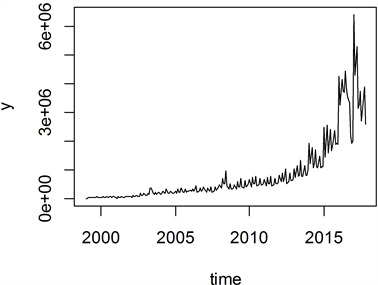Figure 1. Time series chart of China’s health insurance premium income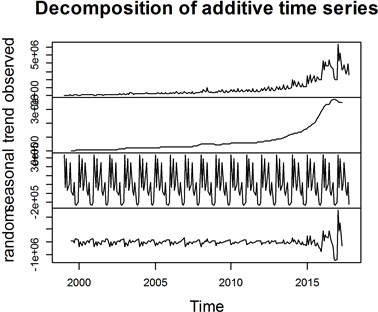Figure 2. Data decomposition map of China’s health insurance premium income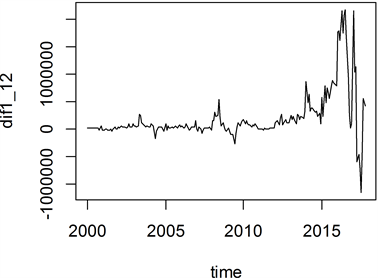Figure 3. Time series diagram of the 1st order 12-step difference graph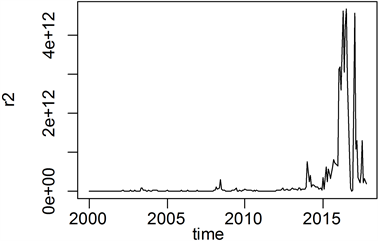Figure 4. 1st order 12-step difference residual squared graph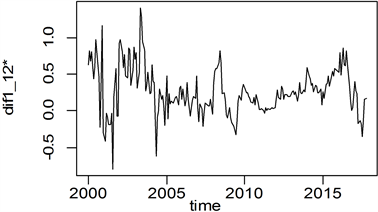Figure 5. Time-series sequence of 1st order 12-step difference after logarithmic transformation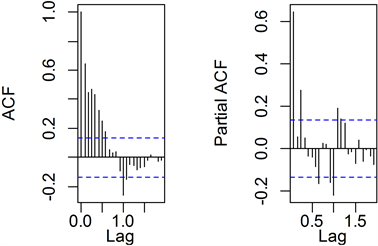Figure 6. Autocorrelation coefficient and partial autocorrelation coefficient of pre-processed sequence

2.2. 平稳序列的白噪声检验Table 1. Parameter table of white noise test

2.3. 模型识别Table 2. AIC values for each valid model

${y}_{t}={\beta }_{0}+{\beta }_{1}{y}_{t-1}+{\beta }_{2}{y}_{t-2}+{\beta }_{3}{y}_{t-3}+{\epsilon }_{t}$ . (2-1)

${y}_{t}=0.06052+0.57761{y}_{t-1}-0.1085{y}_{t-2}+0.28558{y}_{t-3}+{\epsilon }_{t}$ . (2-2)Table 3. Results of the t-test of the parameters

2.4. 分位数回归Table 4. Estimates of coefficients of models corresponding to different quantiles

${Q}_{0.5}\left({y}_{t}|{F}_{t-1}\right)=0.03036+0.70617{y}_{t-1}-0.03864{y}_{t-2}+0.18706{y}_{t-3}$ . (2-3)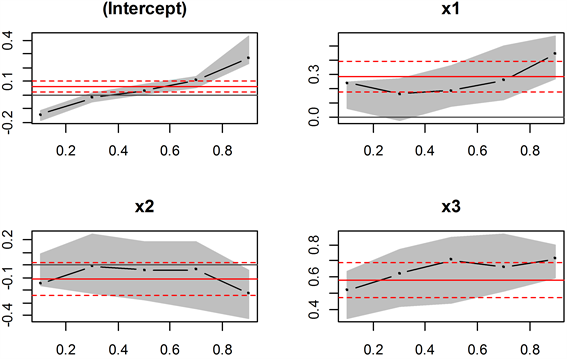Figure 7. Trends and confidence intervals for model parameters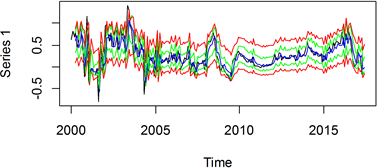Figure 8. Quantile autoregressive model fitting effect diagram

2.5. 序列预测

Table 5. Predicted value of health insurance premium income (ten thousand yuan)

1) 根均方误差 $\text{RMSE}\left(\tau \right)$

$\text{RMSE}\left(\tau \right)=\sqrt{\frac{1}{T}\underset{t=1}{\overset{T}{\sum }}{\left({y}_{t}-{\stackrel{^}{Q}}_{\tau }\left({y}_{t}|{F}_{t-1}\right)\right)}^{2}}$(2-4)

2) 平均绝对误差 $\text{MAE}\left(\tau \right)$

$\text{MAE}\left(\tau \right)=\frac{1}{T}\underset{t=1}{\overset{T}{\sum }}|{y}_{t}-{\stackrel{^}{Q}}_{\tau }\left({y}_{t}|{F}_{t-1}\right)|$ . (2-5)

3) 平均百分比误差 $\text{MAPE}\left(\tau \right)$

$\text{MAPE}\left(\tau \right)=\frac{1}{T}\underset{t=1}{\overset{T}{\sum }}|\frac{{y}_{t}-{\stackrel{^}{Q}}_{\tau }\left({y}_{t}|{F}_{t-1}\right)}{{y}_{t}}|×100%$ . (2-6)Table 6. Comparison of point prediction results

$\text{FICP}\left(\tau \right)=\frac{1}{T}\underset{t=1}{\overset{T}{\sum }}{I}_{t}\left(\tau \right)×100%,\text{\hspace{0.17em}}\tau \in \left(0,0.5\right)$ . (2-7)

3. 结论

NOTES

*通讯作者

 Roger, K. and Bassett, G. (1978) Regression Quantiles. Econometrica, 64.

 朱平芳, 张征宇. 无条件分位数回归: 文献综述与应用实例[J]. 统计研究, 2012, 29(3): 88-96.

 彭良玉. 分位数回归在时间序列中的应用[D]: [硕士学位论文]. 天津: 天津大学, 2010.

 盛选义, 彭良玉. 分位数回归在时间序列中的应用[J]. 太原师范学院学报(自然科学版), 2011, 10(3): 25-29.

 崔丙维. 基于分位数回归的时间序列模型及应用[D]: [硕士学位论文]. 北京: 华北电力大学, 2013.

 国泰安SCMAR数据库. 数据中心[EB/OL].
http://www.gtarsc.com/Home

 杜艳芳. 基于分位数回归的空气质量指数分析[D]: [硕士学位论文]. 兰州: 兰州大学, 2016.

Top